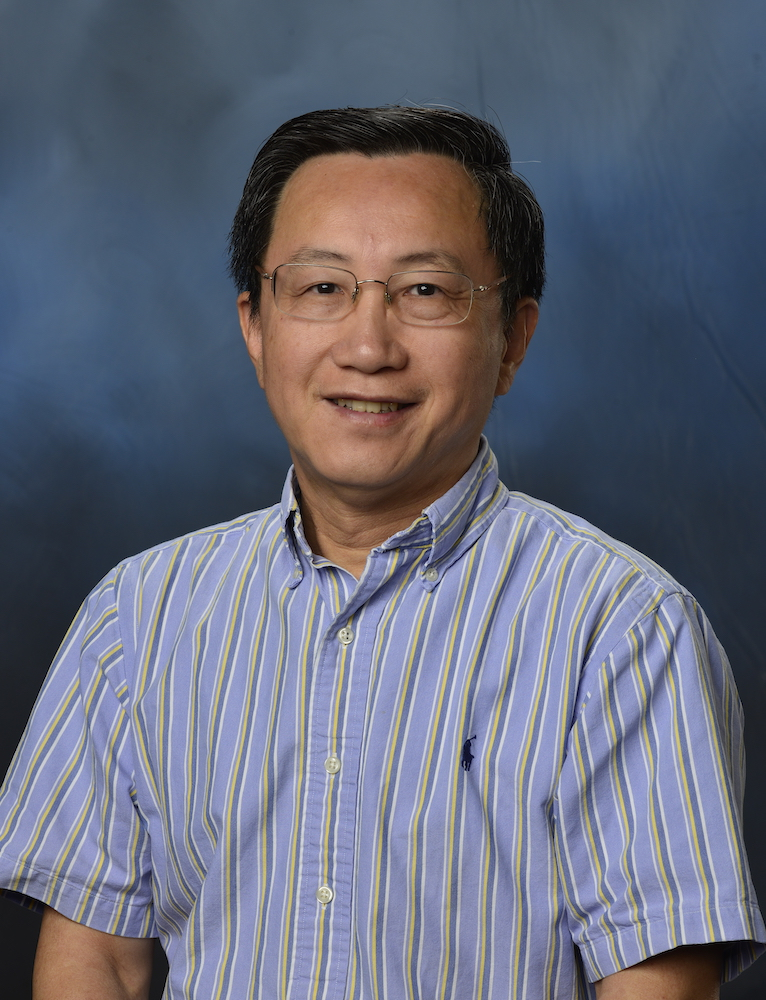COSAM » Events » 2021 » September » DMS Statistics and Data Science Seminar

 DMS Statistics and Data Science Seminar Time: Sep 23, 2021 (02:00 PM) Location: ZOOM Details:Speaker: Yanzhao Cao, Auburn University Title: Uncertainty quantification of deep neural networks   Abstract: Uncertainty quantification of deep neural networks (DNN) is a very important issue in deep learning.  In our UQ for DNN framework, the DNN architecture is the neural ordinary differential equations (Neural-ODE), which formulates the evolution of potentially huge hidden layers in the DNN as a discretized ordinary differential equation (ODE) system. To characterize the randomness caused by the uncertainty of models and noises of data, we add a multiplicative Brownian motion noise to the ODE as a stochastic diffusion term, which changes the ODE to a stochastic differential equation (SDE), and the deterministic DNN becomes a stochastic neural network (SNN). In the SNN, the drift parameters serve as the prediction of the network, and the stochastic diffusion governs the randomness of network output, which serves to quantify the epistemic uncertainty of deep learning. I will present results on convergence as well as numerical experiments.

Last updated: 09/22/2021